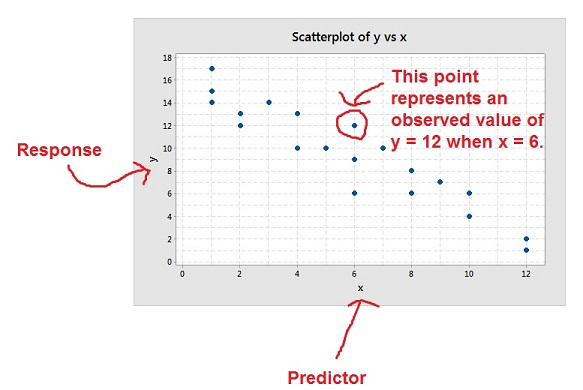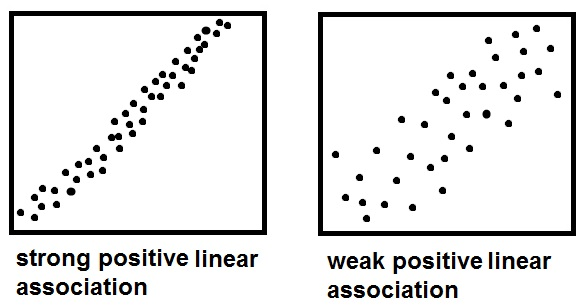﻿ Reading scatterplots - MathBootCamps

Scatterplots are used to understand the relationship or association between two variables. Questions like “When the temperature increases, do gas prices also increase?” or “How are changes in the price of gas related to the number of miles people drive each month?” can be answered by studying the pattern in a scatterplot.

## Basic Structure

Given a scatterplot, the variable on the horizontal axis is the predictor (or independent variable) and the variable on the vertical axis is the response (or dependent variable). Using this terminology, a scatterplot is used to understand how the response responds to changes in the predictor. Each point represents the value of the response for a given value of the predictor. These are called observed values.## Patterns

In general, you can categorize the pattern in a scatterplot as either linear or nonlinear. Scatterplots with a linear pattern have points that seem to generally fall along a line while nonlinear patterns seem to follow along some curve. Whatever the pattern is, we use this to describe the association between the variables. If there is no clear pattern, then it means there is no clear association or relationship between the variables that we are studying.As you can see above, linear patterns can be thought of as either positive or negative. In a positive pattern, as the value of the predictor increases, so does the value of the response. This shows up in the scatterplot as a linear pattern that rises from left to right. In a negative pattern, as the predictor increases, the value of the response decreases. This is seen as a linear pattern that falls from left to right.

## Strength

The strength of the relationship or association between two variables is shown by how close the points are to each other. This is true whether the pattern is linear, nonlinear, positive, or negative.It can be somewhat subjective to compare the strength of one association to another. For scatterplots with linear patterns, the correlation coefficient can be used to better understand this strength.

## Example

Caitlyn has started a business selling textbooks and novels online. In order to better predict her costs, she has been collecting data on the number of books in each shipment she has sent and the weight of the shipment. She plotted the data in the scatterplot below.Use this scatterplot to answer the following questions.
(a) What has Caitlyn used as the predictor? What about the response?
(b) Describe the association between the two variables. Is it weak, strong, or neither?
(c) What was the heaviest shipment Caitlyn made?
(d) One of the shipments weight a little less than 7 pounds. How many books were in this shipment?

### (a) What has Caitlyn used as the predictor? What about the response?

Remember that when using a scatterplot, the idea is to understand how the response responds to changes in the predictor. The predictor is always plotted along the horizontal axis and the response along the vertical. Here, the predictor is the number of books and the response is the weight of the shipment.

### (b) Describe the association between the two variables. Is it weak, strong, or neither?

This is a positive linear pattern. That means that there is a positive association between the number of books in each shipment and the weight. That is, as the number of books increases, the weight of the shipment also increases. The points aren’t that close together, nor are they that far apart so this pattern isn’t really weak or strong.

### (c) What was the heaviest shipment Caitlyn made?

Since the weight was the response, we are looking for the point with the largest value on the vertical axis.From this picture, you can see that the heaviest shipment was 8 pounds.

### (d) One of the shipments weight a little less than 7 pounds. How many books were in this shipment?There is only one shipment with a weight just below 7 pounds. This shipment contained 5 books.

Now that you have seen how to read a scatterplot, the natural question may be “How do I make a scatterplot using a dataset?”. You can click this link to learn how to get a quick scatterplot using your TI83 or TI84 calculator: Making a scatterplot with your graphing calculator.## Subscribe to our Newsletter!

We are always posting new free lessons and adding more study guides, calculator guides, and problem packs.

Sign up to get occasional emails (once every couple or three weeks) letting you know what's new!